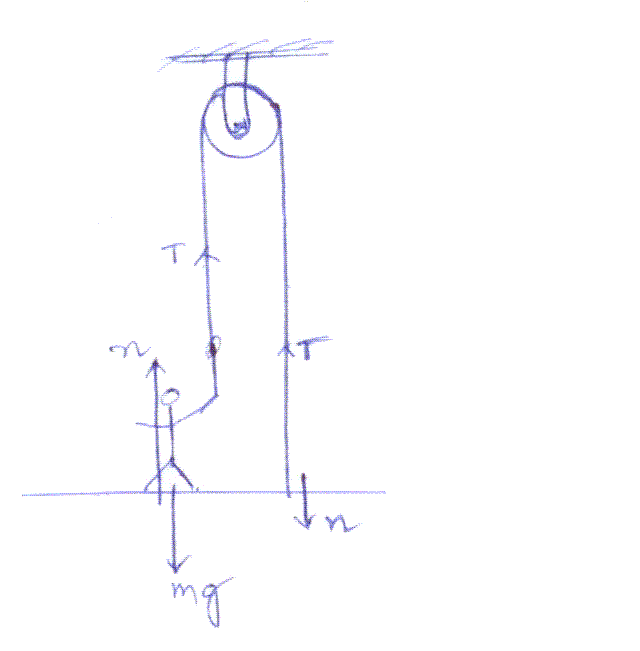Question# A man with mass 70.0 kg stands on a platform withmass 25.0 kg. He pulls on the free end of a rope thatruns over a pulley on the ceiling and has its ot

Other
ANSWEREDA man with mass 70.0 kg stands on a platform withmass 25.0 kg. He pulls on the free end of a rope thatruns over a pulley on the ceiling and has its other end fastened tothe platform. The mass of the rope and the mass of the pulley canbe neglected, and the pulley is frictionless. The rope is verticalon either side of the pulley.
a) With what force does he have to pull so that he and theplatform have an upward acceleration of $$\displaystyle{1.80}\frac{{m}}{{s}^{{2}}}$$?
b) What is the acceleration of the rope relative to him?2021-03-26Part(a)
Equation of motion for the man on the platform is T + n - Mg =Ma
Equation of motion for the platform is T - n - mg =ma
add the above two equations to eliminate n then we will get
$$\displaystyle{T}={\frac{{{\left({M}+{m}\right)}{\left({a}+{g}\right)}}}{{{2}}}}$$
Part(b)
acceleration of the rope = -2 x acceleration of theplatform
$$\displaystyle{a}_{{{r}{o}{p}{e}}}=-{2}\times{1.80}=-{3.6}\frac{{m}}{{s}^{{2}}}$$
$$\displaystyle{a}_{{{r}{o}{p}{e}}}$$ with respect to man is $$\displaystyle=-{3.6}-{1.8}=-{5.4}\frac{{m}}{{s}^{{2}}}$$